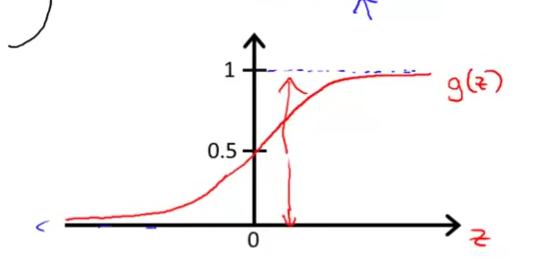# Stanford Machine Learning Note

## 单变量线性回归

### 梯度下降法

• 从任意一个点$(\theta_0 ,\theta_1)$开始，可以取$(0,0)$
• 改变$\theta_0 ,\theta_1$的值，使$J(\theta_0, \theta_1)$越来越小，直到收敛

## 多变量线性回归

### 梯度下降法

$\mu_i$是这个特征的均值，这个处理出来的范围是$[-0.5,0.5]$

### Normal Equation

• 在matlib和Octave里可以使用pinv($X^TX$)求解伪逆矩阵
• 去掉样本中冗余的特征(比如$x_1$$x_2$线性相关)
• 如果$m\leq n$的话，去掉一些特征

### 对比

• 优点：$n$特别大的时候效率很高
• 缺点：需要自己选择$\alpha$;需要多次迭代

Normal Equation：

• 优点：不需要选择$\alpha$;不需要迭代
• 缺点：需要计算$(X^TX)^{-1}$比较花时间；$n$比较大的时候很慢

## Logistic Regression

### Hypothesis Representation### Decision Boundary

• $\theta^Tx>0$的时候，$h_\theta(x)>0.5$$y=1$
• $% $的时候，$% $$y=0$

### Simplified Cost Function and Gradient Descent

$J(\theta)$$Cost(h_\theta(x),y)$和上面的一样，而且对于$y$的值，要么是0要么1,现在对$Cost(h_\theta(x),y)$进行简化：

• BFGS
• L-BFGS